# Generating Reflectance Curves from sRGB Triplets (Page 2)

#### sRGB Triplet from a Reflectance Curve

The reverse process of computing an sRGB triplet from a reflectance curve is straightforward. It requires two main components: (1) a mathematical model of the “standard observer,” which is an empirical mathematical relationship between color stimuli and color sensation (tristimulus values), and (2) a definition of the sRGB color space that specifies the reference illuminant and the mathematical transformation between tristimulus values and sRGB values.

The linear transformation relating a color stimulus,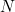, to its corresponding tristimulus values,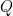, is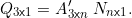The column vectorhas three elements,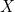,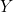, and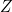. The matrix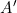has three rows (called the three “color matching functions”) and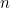columns, whereis the number of discretized wavelength bands. In this study, all computations are performed with 36 wavelength bands of width 10 nm, running over the range 380 nm to 730 nm. The stimulus vector also hascomponents, representing the total power of all wavelengths within each band. The specific color matching functions I use in this work are the CIE 1931 color matching functions. (Note that I’m using the symbolhere; the standard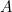matrix isx 3, and soindicates that it has been transposed.)

The stimulus vector can be constructed as the product of an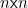diagonal illuminant matrix,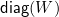, and an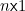reflectance vector,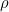. The computation ofis usually normalized so thetristimulus value is equal to 1 whenis a perfect reflector (contains all 1s). The normalizing factor,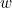, is thus the inner product of the second row ofand the illuminant vector,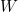, yielding the alternate form of the tristimulus value equation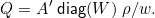The transformation from tristimulus values to sRGB is a two-step process. First, a 3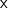3 linear transformation,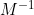, is applied to convertto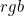, which is a triplet of “linear RGB” values: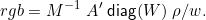The second step is to apply a “gamma correction” to the linearvalues, also known as “companding” or applying the “color component transfer function.” This is how it is done: for each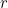,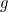, andcomponent of, let’s generically call it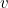, if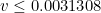, use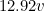, otherwise use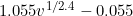. This gives sRGB values in the range of 0 to 1. To convert them to the alternate integer range of 0 to 255 (used in most 24-bit color devices), we multiply each by 255 and round to the nearest integer.

sRGB to rgb
The inverse operation of converting sRGB to, expressed in code, is:
sRGB=sRGB/255; % convert 0-255 range to 0-1 range for i=1:3   if sRGB(i)<0.04045     rgb(i)=sRGB(i)/12.92;   else     rgb(i)=((sRGB(i)+0.055)/1.055)^2.4;   end end

The expression relatingandabove can be simplified by combining the three matrices and the normalizing factor into a single matrix,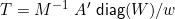so that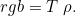The formal definition of the sRGB color space uses an illuminant similar to daylight, called D65, as its “reference” illuminant. Here are the specific values for thematrix, thematrix, the D65vector, and the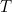matrix. The normalizing factor,, has a value of 10.5677. Most of the RGB-related theory I present here I learned from Bruce Lindbloom’s highly informative website.

Now that we have a simple expression for computing sRGB from a reflectance curve, we can use that as the basis of doing the opposite, computing a reflectance curve from an sRGB triplet. In the sections that follow, I will present five different algorithms for doing this. Each has its strengths and weaknesses. Once they are presented, I will then compare them to each other and to reflectance curves found in nature.